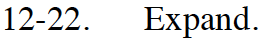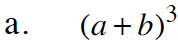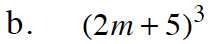Home > CCA2 > Chapter 12 > Lesson 12.1.1 > Problem12-22

12-22.
1. Expand. Homework Help ✎

1. (a + b)3

2. (2m + 5)3Review the Math Notes on the Binomial Theorem in lesson 10.3.1. Remember, you can also use Pascal's Triangle to help you here.

(a + b)3 = 3C3a3b0 + 3C2a2b1 + 3C1a1b2 + 3C0a0b3

a3 + 3a2b + 3ab2 + b3Use the result of part (a). a = 2m; b = 5. Simplify.